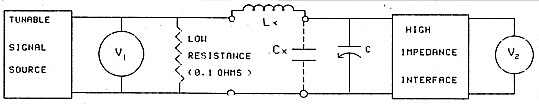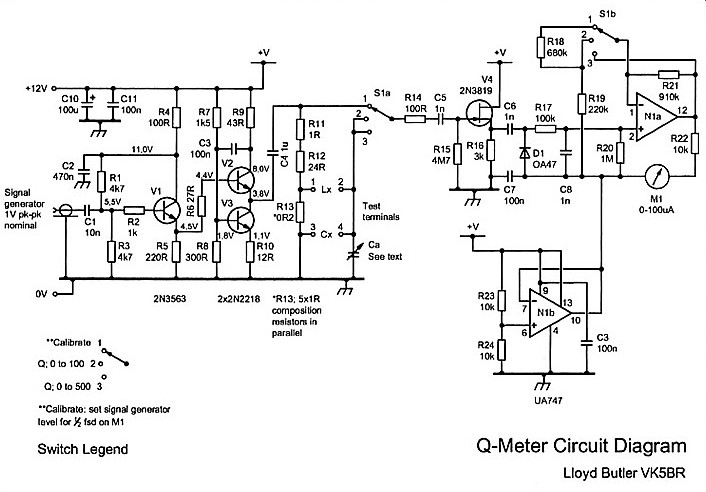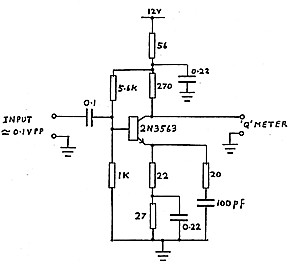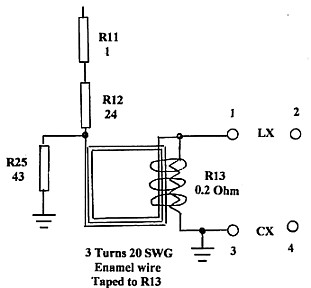QQQ AN EXPERIMENTAL "Q" METER QQQ
by Lloyd Butler VK5BR

(Originally published in Amateur Radio, November 1988)
(Revised April 2004)

The article describes Q factor, methods of measuring Q factor, inductance and capacity using a Q meter and the design of an experimental Q meter unit.

INTRODUCTION

For many years, the Q meter has been an essential piece of equipment for laboratories engaged in the testing of radio frequency circuits. In modem laboratories, the Q meter has been largely replaced by more exotic (and more expensive) impedance measuring devices and today, it is difficult to find a manufacturer who still makes a Q meter. For the radio amateur, the Q meter is still a very useful piece of test equipment and the writer has given some thought to how a simple Q meter could be made for the radio shack. For those who are unfamiliar with this type of instrument, a few introductory notes on the definition of Q and the measurement of Q, are included.

WHAT IS Q AND NOW IS IT MEASURED?

The Q factor or quality factor of an inductance is commonly expressed as the ratio of its series reactance to its series resistance. We can also express the Q factor of a capacitance as the ratio of its series reactance to its series resistance although capacitors are generally specified by the D or dissipation factor which is the reciprocal of Q.

A tuned circuit, at resonance, is considered to have a Q factor. In this case, Q is equal to the ratio of either the inductive reactance, or the capacitive reactance, to the total series loss resistance in the tuned circuit. The greater the loss resistance and the lower the Q, the greater the power lost on each cycle of oscillation in the tuned circuit and hence the greater the power needed to maintain oscillation.

Another way to derive Q is as follows:

Q = fo/Δf   where fo is the resonant frequency   and Δf is the 3 dB bandwidth
(See Footnote on how this is done.)

Sometimes we talk of loaded Q (such as in transmitter tank circuits) and, in this case, resistance for calculation of Q is the unloaded tuned circuit series resistance plus the additional loss resistance reflected in series into the circuit from its coupled load.

There are other ways of expressing Q factor. It can be expressed approximately as the ratio of equivalent shunt resistance to either the inductive or the capacitive reactance. Series loss resistance can be converted to an equivalent shunt resistance using the following formula:

R(shunt) = R (series). (Q² + 1)

Finally, Q factor of a resonant circuit is equal to its voltage magnification factor and Q can also be expressed as the ratio of voltage developed across its reactive elements to the voltage injected in series with the circuit to produce the developed voltage. To measure Q factor, Q meters make use of this principle.

A basic Q meter is shown in Figure 1. Terminals are provided to connect the inductance (Lx) to be measured and this is resonated by a variable tuning capacitor (C). Terminals are also provided to add capacitance (Cx), if required. The tuned circuit is excited from a tunable signal source which develops voltage across a resistor in series with the tuned circuit. The resistor must have a resistance small compared to the loss resistance of the components to be measured so that its value can be ignored. A resistance of a mere fraction of an ohm is necessary. Metering is provided to measure the AC injection voltage across the series resistor and the AC output voltage across the terminals of the tuning capacitor. The output measurement must be a high input impedance circuit to prevent loading of the tuned circuit by the metering circuit.Figure 1 - Basic Q Meter
At resonance of Lx and Cx, Q = V2/V1
*Meter V2 is Calibrated to read voltage referred to that across C.

Q is measured by adjusting the source frequency and/or the tuning capacitor for a peak in output voltage corresponding to resonance. Q factor is calculated as the ratio of output voltage measured across the tuned circuit to that injected into it. In practice, the signal source level is generally set for a calibrate point on the meter which measures injected voltage and Q is directly read from calibration on the meter which measures output voltage.

SOME USES OF THE Q METER

The Q meter can be used for many purposes. As the name implies, it can measure Q and is generally used to check the Q factor of inductors. As the internal tuning capacitor has an air dielectric its loss resistance is negligible compared to that of any inductor and hence the Q measured is that of the inductor.

The value of Q varies considerable with different types of inductors used over different ranges of frequency. Miniature commercial inductors, such as the Siemens B78108 types or the Lenox-Fugal Nanored types, made on ferrite cores and operated at frequencies up to 1 MHz, have typical Q factors in the region of 50 to 100. Air wound inductors with spaced turns, such as found in transmitter tank circuits and operating at frequencies above 10 MHz, can be expected to have Q factors of around 200 to 500. Some inductors have Q factors as low as five or 10 at some frequencies and such inductors are generally unsuitable for use in selective circuits or in sharp filters. The Q meter is very useful to check these out.

The tuning capacitor (C) of the Q meter has a calibrated dial marked in pico-farads so that, in conjunction with the calibration of the oscillator source, the value of inductance (Lx) can be derived. The tuned circuit is simply set to resonance by adjusting the frequency and/or the tuning capacitor for a peak in the output voltage meter and then calculating the inductance (Lx) from the usual formula:

Lx = 1/4π²f²C

For L in μH, C in pF and f in MHz this reduces to:

25330/f²C

Another use of the Q meter is to measure the value of small capacitors. Providing the capacitor to be tested is smaller than the tuning range of the internal tuning capacitor, the test sample can be easily measured. Firstly, the capacitor sample is resonated with a selected inductor by adjusting the source frequency and using the tuning capacitor set to a low value on its calibrated scale. The sample is then disconnected and using the same frequency as before, the tuning capacitor is reset to again obtain resonance. The difference in tuning capacitor calibration read for the two tests is equal to the capacitance of the sample. Larger values of capacitance can be read by changing frequency to obtain resonance on the second test and manipulating the resonance formula.

A poorly chosen inductor is not the only cause of low Q in a tuned circuit as some types of capacitor also have high loss resistance which lowers the Q. Small ceramic capacitors are often used in tuned circuits and many of these have high loss resistance, varying considerably in samples often taken from the same batch. If ceramic capacitors must be used where high Q is required, it is wise to select them for low loss resistance and the Q meter can be used for this purpose. To do this, an inductor having a high Q, of at least 200, is used to resonate the circuit, first with the tuning capacitor (C) on its own and then with individual test sample capacitors in parallel. A drastic loss in the value of Q, when the sample is added, soon shows up which capacitor should not be used.

DISTRIBUTED COIL CAPACITANCE

Direct measurement of Q in an inductor, as discussed in previous paragraphs. is based on the circuit having two components, inductance and capacitance. Inductors also have distributed capacitance (Cd) and if this represents a significant portion of the total tuning capacitance, the Q value read will be lower than its actual value. High distributed capacitance is common in large value inductors having closely wound turns or having multiple layers.

Actual Q can be calculated from Qe, as read, from the following:

Q = Qe (1 + Cd/C)
where Cd = Distributed capacitance
and C = Tuning Capacitance

Q value error is reduced by resonating with a large value of tuning capacitance, otherwise distributed capacitance can be measured and applied to the previous formula. Two methods of measuring distributed capacitance are described in the "Boonton Q Meter Handbook". The simplest of these is said to be accurate for distributed capacitance above 10 pF and this method is described as follows:

1. With the tuning capacitor (C) set to value C1 (say 50 pF), resonate with the sample inductor by adjusting the signal source frequency.

2. Set the signal source to half the original frequency and re-resonate by adjusting C to a new value of capacitance C2.

3. Calculate distributed capacitance as follows:   Cd = (C2 -4C1) /3

Another effect of distributed capacitance in the inductor is to make its inductance value (as calculated from the calibration of the tuning capacitance and the calibration of the signal source) appear higher than its actual value. Again, this error can be reduced by tuning with a large value of capacitance C and/or adding Cd to C in the calculation.

THE EXPERIMENTAL UNIT

A little circuit design and experimentation have led to the Q meter circuit shown in Figure 2. A signal source is not included as it was thought that most experimenters would have some type of signal generator which could be used as a source. Addition of a signal source, normally internal in a commercial Q meter, would have added complexity which it was decided to avoid at the initial stage.Figure 2 - Q Meter Circuit Diagram.
Test terminals 1-4 are to connect Lx and Cx.
R13 (0.2 Ω) is five 1 Ω composition resistors in parallel.
To Calibrate, set Sig Gen level for half scale on M1.

In designing a circuit, the biggest problem seemed to be how to develop an easily measured source voltage with a source resistance of a mere fraction of an ohm. An initial thought was to use a small multi-filar wound toroidal step down transformer from a higher resistance source. (In such a transformer, coefficient of coupling is high and leakage inductance is low). For this application, leakage inductance reflected in series with the secondary proved to be still too high and the idea was abandoned.

Another idea was to make use of the low source resistance of a power voltage follower stage to directly inject a signal into the tuned circuit. The follower circuit shown as V2-V3 in Figure 2 was used for this purpose. This type of circuit has wide bandwidth with very low source resistance and has been used before for such purposes as driving video signals into a low impedance transmission line. For the Q meter case, it was found necessary to operate the stage at the high collector current of 100 mA to achieve a sufficiently low source resistance. Because of this, transistors V2 and V3 ran with their TO5 cases quite hot. The circuit worked well at low frequencies but at higher frequencies, in the region of 10 to 30 MHz, the stage source resistance appeared to rise causing the Q values to read lower than expected.

The ultimate circuit, as shown in Figure 2, made use of the voltage follower stage but the stage was used to develop voltage across R13, a resistor of a fraction of an ohm as described in previous paragraphs. The value actually chosen is 0.2 ohm. Of course, the follower stage could not drive directly into such a low resistance and it feeds via resistors R11 and R12 (a sum of 25 ohms) so that the follower output voltage is 125 times that injected into the tuned circuit.

The power driver stage is preceded by an emitter follower stage (V1). This has high input resistance and hence the load resistance presented to the external signal source is essentially the parallel result of Rl and R3 (about 2300 ohms).

The test inductance (Lx) is connected across terminals 1 and 2 and external capacitance (Cx), if used, is connected across terminals 3 and 4. Tuning is provided by variable capacitor Ca, an ordinary receiver tuning gang with sections in parallel to provide about 800 pF maximum capacitance.

The high impedance voltmeter is provided by FET stage V4, connected as a source follower, peak reading detector (C6, D1, R17, C8, R20) and operational amplifier N1-A which drives a 100 microamp meter. The second operational amplifier NI-B in the uA747 package splits the rail voltage for N1-A.

Selector switch (S1) has three positions. The first position, labelled CAL, is used to set the signal input level which is adjusted for half scale reading on meter M1. (A signal level of around 1 VPP is needed at the input of V1). When the signal is set to the correct level, switch position 2 provides direct reading of Q = 0 to 100 on the meter and switch position 3 provides direct reading of Q = 0 to 500 on the meter. For low values of Q, the calibrate level at switch position 1 is increased to full scale on the meter so that switch position 2 reads 0 = 0 to 50.

Signal levels into the AC volt-meter circuit are proportioned so that they are above the nonlinear region caused by the diode characteristics, but within the limits of signal voltage swing set by the power supply rails. In switch position 1 (CAL) the amplifier N1-A has a voltage gain of 2, in switch position 2 it has a gain of 5 and in switch position 3 it has a gain of 1.

A rail potential of 12 volts was selected to power the unit but its precise value is not critical. The supply current is quite high, at around 100 mA, because of the current consumed by the V2-V3 transistor follower stage.

PERFORMANCE

Comparing Q readings with those measured on other instruments, the experimental unit appears sufficiently accurate to assess the performance of most inductors around the radio shack. For very high values of Q (around 400), with Ca set to minimum, the Q reads a trifle low. This occurs because of loss in resistor R14 connected in series with the input capacitance of V4. (The reading can be improved by eliminating R14, but without it, V4 is inclined to be unstable when Ca is bridged directly across its input). For a higher setting of capacitance Ca, the input capacitance of V4 is masked and the Q error is less noticeable.

Accuracy of inductance and capacitance measurement is set by the accuracy of the signal source and the accuracy of the tuning capacitor dial calibration. For anyone interested in building the Q meter, the calibration of the dial can be carried out by direct measurement of the tuning capacitor, at various dial settings, using a capacitance bridge or another Q meter. Another method is to make use of the signal source calibration in conjunction with an inductor of known accurate value. For various settings of the tuning capacitor dial, the signal source frequency is set for indicated resonance in the Q meter and the value of capacitance calculated. Assuming the inductor value and signal source frequency to be accurate, this is probably the best method as it takes into account added wiring capacitance and the active input capacitance of V4.

The unit was found to work quite well over the frequency range of 100 kHz to 40 MHz. Attempts to tune above 40 MHz gave erratic results but operation into the VHF range had never really been anticipated.

NOTES ON ASSEMBLY

The circuit shown in Figure 2 is simple and should not be too difficult to duplicate. All resistors in the RF sections of the circuit must be carbon types with low inductance. Resistor R13 (0.2 ohm) is made up of five one ohm resistors connected in parallel. The RF drive circuit (V1, V2, V3) is separated from the detector circuit (V4, D1, N1) by fitting on separate cards which are kept apart to reduce stray coupling between them. RF inter-wiring into the test terminals, capacitor Ca and switch bank S1a is kept direct and short and must not be loomed. Resistor R13 is mounted directly on the test terminals. Terminal 4 is directly earthed to chassis and Ca is strapped to terminals 3 and 4 with a short length of tinned copper wire to minimise series inductance.

Transistors V2-V3 (type 2N2218) have a cut off frequency of 250 MHz and can dissipate 680 mW at 50 degrees Celsius. These could be substituted with other transistors of similar characteristics. Likewise, transistors V1 (2N3563) and V4 (FET - 2N3819) could be substituted with other small signal transistors having a high cut off frequency.

SUMMARY

A few ideas have been presented on how a simple Q meter can be built and how it can be put to use. Other applications of the Q meter can be found in manuals on early Q meters such as that prepared by the Boonton Radio Corporation'.

REFERENCES

1. Manual of Radio Frequency Measurements for the Q Meter. Boonton Radio Corporation.

ADDENDUM - A SIGNAL SOURCE PRE-AMPLIFIER

The original experimental Q meter (as described above) required an external RF signal source of around one volt PP. Not all signal generators can deliver quite this level of output voltage and for use with these generators, some pre-amplification is needed at the Q meter signal input.Figure 3 Q Meter Preamplifier. 100 kHz to 40 MHz.

The wideband amplifier shown in figure 3 provides a gain of approximately 10 over the operating range of the Q meter of 100 kHz to 40 MHz. Connected at the input of the Q meter, it changes the input sensitivity to about 0.1 VPP to make the Q meter usable with a greater variety of RF signal sources. No gain control is provided as signal generators normally have an adjustable attenuator to set the output level.

For those who might be considering duplicating the Q meter, the pre-amplifier is a useful addition to make it operate in conjunction with lower level signal generators.

MODIFICATIONS TO RF DIVIDER CIRCUIT

A divider network in the original circuit of figure 1 consists of R11, R12 and R13. This divides the RF voltage by 125 so that the voltage across R13 (0.2 ohm) is 1/125 of that fed from the power amplifier. This works fine at low frequencies but as the frequency is increased, the division factor is reduced making the Q measurement look higher than it actually is.

The explanation of this is as follows: The circuit from terminal 1 through R13 to terminal 3 is a short conductor which has finite inductance. If we assume that conductor is 5 cm long, it must have an inductance of around 0.02 uH to 0.03 uH depending on its diameter. Whilst this is a very small inductance, its reactance could be as high as 1 ohm at frequencies around 6 to 8 MHz. Clearly such a high reactance developed in series with the 0.2 ohm resistance of R13 increases the proportion of voltage across terminals 1 and 3 as frequency is increased.

To cancel this effect, the circuit modification shown in figure 4 has been devised. The idea is to develop a reverse field around R13 from the current flowing into it so that the offending inductance is cancelled. To get enough field, three series connected conductors carrying the input current are taped to the five parallel resistors making up the 0.2 ohm R13.

A further addition is R25, 43 ohms. The looped wires around R13 form a coil and R43 is added to lower the Q of that coil and prevent instability around the amplifier chain which occurs without the R25 addition.

In the Q meter tested, the division ratio was held quite constant up to 40 MHz with a few small perturbations around 20 to 30 MHz region. The modificaton provides a considerable improvement in the accuracy of direct Q measurement.Figure 4
Modifications to RF Divider Circuit

LATER FOOTNOTE from Writer

The Q Meter is still in use but where accuracy is need in Q measurement, I connect a frequency counter across the signal generator to measure precise frequency. The tuned circuit is resonated and M1 adjusted for full scale reading by adjusting the level from the signal generator. The frequency is then adjusted either side of resonance for a reading on M1 of 0.7 of the full scale reading and the two side frequencies as read on the counter are recorded. The ratio of the center frequency to the difference between the two side frequencies is then calculated as Q.